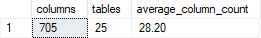## Find number of columns in SQL Server database

Article for:

Query returns basic statistics of numbers of columns in a database.

## Query

``````select [columns],
[tables],
CONVERT(DECIMAL(10,2),1.0*[columns]/[tables]) as average_column_count
from (
select count(*) [columns],
count(distinct schema_name(tab.schema_id) + tab.name) as [tables]
from sys.tables as tab
inner join sys.columns as col
on tab.object_id = col.object_id
) q
``````

## Columns

• columns - total number of columns in a database
• tables - number of tables in a database
• average_column_count - average number of columns in a table in a database

## Rows

• Query returns just one row

## Sample results